• 在过去的十年中，神经网络取得了巨大的成功。但是，只能使用常规或欧几里得数据来实现神经网络的早期变体，而现实世界中的许多数据都具有非欧几里得的底层图形结构。数据结构的不规则性导致了图神经网络的最新发展。...
• 一个对论文DROPEDGE: TOWARDS DEEP GRAPH CONVOLUTIONAL NETWORKS ON NODE CLASSIFICATION（Dropedge：面向节点分类的deepGCN）进行讲解的原创PPT，有21页。
• Together：通过跨网络深度网络嵌入进行节点分类 该存储库包含作者在 Matlab 中对论文“Network Together: Node Classification via Cross-Network Deep Network Embedding”的实现。 代码： “source_SAE.m”是SAE_s...
•研究论文
• ## GCN节点分类

千次阅读 热门讨论 2020-11-07 12:55:46
通过PyTorch实现GCN用于节点分类
简介
本文通过 Pytorch 实现 GCN 对 Cora 数据集进行节点分类。
数据集说明
Cora 是一个引文关系数据集，由 2708 篇论文以及它们之间的引用关系构成边形成一个图（Graph）。这些论文按照主题被分为了 7 类，分别为神经网络、强化学习、规则学习、概率方法、遗传算法、理论研究和案例相关。每篇论文的特征通过词袋模型获得，维度为 1433，每一维代表一个词，

1

1

表示该词在该文章中出现，

0

0

表示未出现。
数据预处理
首先是下载 Cora 原始数据集，这里由于外网访问的原因，我已经将原始数据集和本文代码处理过的数据集上传到BaiduNetDisk,code:zczc，将该数据集下解压后放到 dataset 目录下即可。
获得原始数据集之后，对其进行处理，得到一个命名元组，该元组包含以下内容：
x: 所有节点的特征，shape为(2708, 1433)
y: 所有节点的label，shape为(2708, )

Cora 数据集由于比较小，这里没有采取分批读取的思路，而是读出来之后再分批取，这样因为没有了本地 IO 会大大提高效率。这部分由于代码比较琐碎，就不列出了，可以通过文末Github访问仓库源码。
GCN 模型
根据GCN的定义

X

′

=

σ

(

L

~

s

y

m

X

W

)

X' = \sigma(\widetilde{L}_{sym} X W)

来定义GCN层，然后堆叠三层GCN层构建图卷积网络，源码如下。
class GraphConvolution(nn.Module):
def __init__(self, input_dim, output_dim, use_bias=True):
"""
L*X*\theta
:param input_dim: 节点输入特征维度
:param output_dim: 输出特征维度
:param use_bias: 是否偏置
"""
super(GraphConvolution, self).__init__()
self.input_dim = input_dim
self.output_dim = output_dim
self.use_bias = use_bias
self.weight = nn.Parameter(torch.Tensor(input_dim, output_dim))
if self.use_bias:
self.bias = nn.Parameter(torch.Tensor(output_dim))
else:
self.register_parameter('bias', None)
self.reset_parameters()

def reset_parameters(self):
init.kaiming_uniform_(self.weight)
if self.use_bias:
init.zeros_(self.bias)

support = torch.mm(input_feature, self.weight)
if self.use_bias:
output += self.bias
return output

def __repr__(self):
return self.__class__.__name__ + ' (' + str(self.input_dim) + ' -> ' + str(self.output_dim) + ')'

class GCN(nn.Module):

def __init__(self, input_dim=1433):
"""
两层GCN模型
:param input_dim: 输入维度
"""
super(GCN, self).__init__()
self.gcn1 = GraphConvolution(input_dim, 128)
self.gcn2 = GraphConvolution(128, 7)

return logits

训练及其可视化
利用Cora数据集进行训练，训练代码如下，由于Cora很小，这里就直接全集进行训练。
from collections import namedtuple

import torch
import torch.nn as nn
import torch.optim as optim
import numpy as np
from sklearn.manifold import TSNE
import matplotlib.pyplot as plt

from model import GCN

# hyper params
LEARNING_RATE = 0.1
WEIGHT_DACAY = 5e-4
EPOCHS = 50
DEVICE = "cuda:0" if torch.cuda.is_available() else "cpu"

# 加载数据，并转换为torch.Tensor
node_feature = dataset.x / dataset.x.sum(1, keepdims=True)  # 归一化数据，使得每一行和为1
tensor_x = torch.from_numpy(node_feature).to(DEVICE)
tensor_y = torch.from_numpy(dataset.y).to(DEVICE)

num_nodes, input_dim = node_feature.shape
tensor_adjacency = torch.sparse.FloatTensor(indices, values, (num_nodes, num_nodes)).to(DEVICE)

# 模型定义：Model, Loss, Optimizer
model = GCN(input_dim).to(DEVICE)
criterion = nn.CrossEntropyLoss().to(DEVICE)

def train():
model.train()
train_loss_history = []
train_acc_history = []
val_acc_history = []
val_loss_history = []
for epoch in range(EPOCHS):
logits = model(tensor_adjacency, tensor_x)  # 前向传播
loss = criterion(train_mask_logits, train_y)  # 计算损失值
loss.backward()
optimizer.step()
train_acc, _, _, train_loss = test(tensor_train_mask, tensor_y)
val_acc, _, _, val_loss = test(tensor_val_mask, tensor_y)
train_loss_history.append(loss.item())
train_acc_history.append(train_acc)
val_loss_history.append(val_loss)
val_acc_history.append(val_acc.item())
print("epoch {:03d}: training loss {:.4f}, training acc {:.4}, validation acc {:.4f}".format(epoch, loss.item(),
train_acc.item(),
val_acc.item()))
return train_loss_history, train_acc_history, val_loss_history, val_acc_history

model.eval()

def plot_loss_with_acc(train_loss_history, train_acc_history, val_loss_history, val_acc_history):
plt.figure(figsize=(12, 8))
plt.plot(range(len(train_loss_history)), train_loss_history, c=np.array([255, 71, 90]) / 255.,
label='training loss')
plt.plot(range(len(val_loss_history)), val_loss_history, c=np.array([120, 80, 90]) / 255.,
label='validation loss')
plt.xlabel('Epoch')
plt.ylabel('Loss')
plt.legend(loc=0)
plt.title('loss')
plt.savefig("../assets/loss.png")
plt.show()

plt.figure(figsize=(12, 8))
plt.plot(range(len(train_acc_history)), train_acc_history, c=np.array([255, 71, 90]) / 255.,
label='training acc')
plt.plot(range(len(val_acc_history)), val_acc_history, c=np.array([120, 80, 90]) / 255.,
label='validation acc')
plt.xlabel('Epoch')
plt.ylabel('Accuracy')
plt.legend(loc=0)
plt.title('accuracy')
plt.savefig("../assets/acc.png")
plt.show()

train_loss, train_acc, val_loss, val_acc = train()
test_acc, test_logits, test_label, _ = test(tensor_test_mask, tensor_y)
print("Test accuarcy: ", test_acc.item())
plot_loss_with_acc(train_loss, train_acc, val_loss, val_acc)

# tsne visualize
tsne = TSNE()
out = tsne.fit_transform(test_logits)
fig = plt.figure()
for i in range(7):
indices = test_label == i
x, y = out[indices].T
plt.scatter(x, y, label=str(i))
plt.legend(loc=0)
plt.savefig('tsne.png')
plt.show()


训练过程的可视化如下面两张图，分别是训练集和验证集上的loss变化和训练集和验证集上的准确率变化。可以看到，训练集损失很快下降且准确率到达1，验证集也很快收敛准确率在0.8左右，这也说明，三层GCN的建模能力虽然不错但也很有限。同时，通过TSNE将输出层的score嵌入进行二维可视化，可以发现，区分度还是很高的，这表示GCN学到的特征其实是很有效的。补充说明
本文数据处理部分思路参考自PyG框架以及《深入浅出图神经网络》的内容，对图网络理论感兴趣的可以阅读这本书。本文涉及到的代码开源于 Github（地址为https://github.com/luanshiyinyang/GNN，如前面的链接跳转有问题，访问该链接即可），欢迎 star 和 fork。
展开全文• 图卷积 节点分类Neural Networks have gained massive success in the last decade. However, early variants of Neural Networks could only be implemented using regular or Euclidean data, while a lot of data...

图卷积 节点分类

Neural Networks have gained massive success in the last decade. However, early variants of Neural Networks could only be implemented using regular or Euclidean data, while a lot of data in the real world have underlying graph structures which are non-Euclidean. The non-regularity of data structures have led to recent advancements in Graph Neural Networks. In the past few years, different variants of Graph Neural Networks are being developed with Graph Convolutional Networks (GCN) being one of them. GCNs are also considered as one of the basic Graph Neural Networks variants.
在过去的十年中，神经网络取得了巨大的成功。 但是，只能使用常规或欧几里得数据来实现神经网络的早期变体，而现实世界中的许多数据具有非欧几里得的底层图形结构。 数据结构的不规则性导致了图神经网络的最新发展。 在过去的几年中，正在开发图神经网络的各种变体，其中之一就是图卷积网络(GCN)。 GCN也被视为基本的Graph Neural Networks变体之一。
In this article, we’ll dive deeper into Graph Convolutional Networks developed by Thomas Kipf and Max Welling. I will also be giving some very basic examples on building our first graph using NetworkX. By the end of this article, I hope we can gain deeper understanding on the mechanisms inside Graph Convolutional Networks.
在本文中，我们将更深入地研究由Thomas Kipf和Max Welling开发的图卷积网络。 我还将在使用NetworkX构建第一个图形时给出一些非常基本的示例。 到本文结尾，我希望我们对图卷积网络内部的机制有更深入的了解。
If you are not familiar with the basic concepts of Graph Neural Networks, I recommend reading my previous article here.
如果您不熟悉Graph Neural Networks的基本概念，建议您 在此处 阅读我的上一篇文章 。

图神经网络中的卷积 (Convolution in Graph Neural Networks)
If you are familiar with convolution layers in Convolutional Neural Networks, ‘convolution’ in GCNs is basically the same operation. It refers to multiplying the input neurons with a set of weights that are commonly known as filters or kernels. The filters act as a sliding window across the whole image and enable CNNs to learn features from neighboring cells. Within the same layer, the same filter will be used throughout image, this is referred to as weight sharing. For example, using CNN to classify images of cats vs non-cats, the same filter will be used in the same layer to detect the nose and the ears of the cat.
如果您熟悉卷积神经网络中的卷积层 ，则GCN中的“卷积”基本上是相同的操作。 它是指将输入神经元乘以一组权重(通常称为过滤器或内核)。 滤镜充当整个图像的滑动窗口，并使CNN能够从相邻单元中学习特征。 在同一层中   在整个图像中将使用相同的过滤器，这称为权重共享 。 例如，使用CNN对猫和非猫的图像进行分类，将在同一层中使用相同的过滤器来检测猫的鼻子和耳朵。
The same weight (or kernel, or filter in CNNs) is applied throughout the image (image by author)

GCNs perform similar operations where the model learns the features by inspecting neighboring nodes. The major difference between CNNs and GNNs is that CNNs are specially built to operate on regular (Euclidean) structured data, while GNNs are the generalized version of CNNs where the numbers of nodes connections vary and the nodes are unordered (irregular on non-Euclidean structured data).
GCN执行类似的操作，其中模型通过检查相邻节点来学习特征。 CNN和GNN之间的主要区别在于，CNN是专门为在常规(欧几里得)结构化数据上运行而构建的，而GNN是CNN的广义版本，其中节点连接的数量变化且节点无序(在非欧几里德结构上是不规则的)数据)。
source
来源图解2D卷积神经网络(左)和图卷积网络(右)

GCNs themselves can be categorized into 2 major algorithms, Spatial Graph Convolutional Networks and Spectral Graph Convolutional Networks. In this article, we will be focusing on Fast Approximation Spectral-based Graph Convolutional Networks.
GCN本身可分为2种主要算法： 空间图卷积网络和频谱图卷积网络 。 在本文中，我们将重点介绍基于快速逼近谱的图卷积网络 。

Before diving into the calculations happening inside GCNs, let’s briefly recap the concept of forward propagation in Neural Networks first. You can skip the following section if you’re familiar with it.
在深入探讨GCN内部发生的计算之前，让我们先简要回顾一下神经网络中的前向传播概念。 如果您熟悉它，可以跳过以下部分。
神经网络正向传播简要回顾 (Neural Networks Forward Propagation Brief Recap)
Illustration of Fully-Connected Neural Networks (image by author)

In Neural Networks, in order to propagate the features representation to the next layer (forward pass), we perform the equation below:
在神经网络中，为了将要素表示传播到下一层(前进)，我们执行以下方程式：
Equation 1 — Forward Pass in Neural Networks

This is basically equivalent to y = mx+b in Linear Regression, where:
这基本上等于线性回归中的 y = mx + b ，其中：
m is equivalent to the weights
m等于权重
x is the input features
x是输入要素
b is the bias
b是偏差
What distinguishes the forward pass equation above from Linear Regression is that Neural Networks apply non-linear activation functions in order to represent the non-linear features in latent dimension.
上面的正向传递方程与线性回归的区别在于，神经网络应用非线性 激活函数来表示潜在维中的非线性特征。
Looking back at the equation above, for the first hidden layer (i = 0), we can simply re-write the equation to be as follows:
回顾上面的方程式，对于第一个隐藏层(i = 0)，我们可以简单地将方程式重写如下：
Equation 2 — Forward Pass in Neural Networks at the first layer

where features representation at layer 0 is basically the input features (X).
其中第0层的要素表示基本上是输入要素(X) 。
How does this equation differ in Graph Convolutional Networks?
这个方程在图卷积网络中有何不同？
快速近似光谱图卷积网络 (Fast Approximate Spectral Graph Convolutional Networks)
The original idea behind Spectral GCN was inspired by signal/wave propagation. We can think of information propagation in Spectral GCN as signal propagation along the nodes. Spectral GCNs make use of the Eigen-decomposition of graph Laplacian matrix to implement this method of information propagation. To put it simply, the Eigen-decomposition helps us understand the graph structure, hence, classifying the nodes of the graphs. This is somewhat similar to the basic concept of Principal Component Analysis (PCA) and Linear Discriminant Analysis (LDA) where we use Eigen-decomposition to reduce dimensionality and perform clustering. If you have never heard of Eigen-decomposition and Laplacian matrix, don’t worry! In this Fast Approximation method, we are not going to use them explicitly.
频谱GCN背后的原始想法是受信号/波传播的启发。 我们可以将频谱GCN中的信息传播视为沿节点的信号传播。 频谱GCN利用图拉普拉斯矩阵的特征分解来实现这种信息传播方法。 简而言之，本征分解可帮助我们理解图的结构，从而对图的节点进行分类。 这有点类似于主成分分析(PCA)和线性判别分析(LDA)的基本概念，在这些概念中，我们使用特征分解来降低维数并执行聚类。 如果您从未听说过本征分解和拉普拉斯矩阵， 请不用担心！ 在这种快速逼近方法中，我们将不会明确使用它们。
In this approach, we will take into account the Adjacency Matrix (A) in the forward propagation equation in addition to the node features (or so-called input features). A is a matrix that represents the edges or connection between the nodes in the forward propagation equation. The insertion of A in the forward pass equation enables the model to learn the feature representations based on nodes connectivity. For the sake of simplicity, the bias b is omitted. The resulting GCN can be seen as the first-order approximation of Spectral Graph Convolution in the form of a message passing network where the information is propagated along the neighboring nodes within the graph.
在这种方法中，除了节点特征(或所谓的输入特征)外，我们还将考虑前向传播方程中的邻接矩阵(A )。 A是代表前向传播方程中节点之间的边缘或连接的矩阵。 在向前通过方程式中插入A可实现   该模型可基于节点连接性学习特征表示。 为简单起见，偏置b为   省略。 所得的GCN可以看作是消息传递网络形式的频谱图卷积的一阶近似，其中信息沿着图内的相邻节点传播。
通过添加邻接矩阵作为附加元素，前向通过方程将变为：
Equation 3— Forward Pass in Graph Convolutional Networks

Wait.. You said A, what is A*?
等待..您说A，什么是 A * ？
A* is the normalized version of A. To get better understanding on why we need to normalize A and what happens during forward pass in GCNs, let’s do an experiment.
A *是A的规范化版本。 为了更好地理解为什么我们需要对A进行规范化以及GCN中的正向传递过程中会发生什么，我们做一个实验。
建立图卷积网络 (Building Graph Convolutional Networks)
初始化图G (Initializing the Graph G)
Let’s start by building a simple undirected graph (G) using NetworkX. The graph G will consist of 6 nodes and the feature of each node will correspond to that particular node number. For example, node 1 will have a node feature of 1, node 2 will have a node feature of 2, and so on. To simplify, we are not going to assign edge features in this experiment.
让我们开始使用NetworkX构建一个简单的无向图( G )。 图形G将由6个节点组成，每个节点的特征将对应于该特定节点号。 例如，节点1的节点特征为1，节点2的节点特征为2，依此类推。 为简化起见，在本实验中我们将不分配边缘特征。
import networkx as nx
import numpy as np
import matplotlib.pyplot as plt
from scipy.linalg import fractional_matrix_power

import warnings
warnings.filterwarnings("ignore", category=UserWarning)

#Initialize the graph
G = nx.Graph(name='G')

#Create nodes
#In this example, the graph will consist of 6 nodes.
#Each node is assigned node feature which corresponds to the node name
for i in range(6):

#Define the edges and the edges to the graph
edges = [(0,1),(0,2),(1,2),(0,3),(3,4),(3,5),(4,5)]

#See graph info
print('Graph Info:\n', nx.info(G))

#Inspect the node features
print('\nGraph Nodes: ', G.nodes.data())

#Plot the graph
nx.draw(G, with_labels=True, font_weight='bold')
plt.show()
Output:
输出：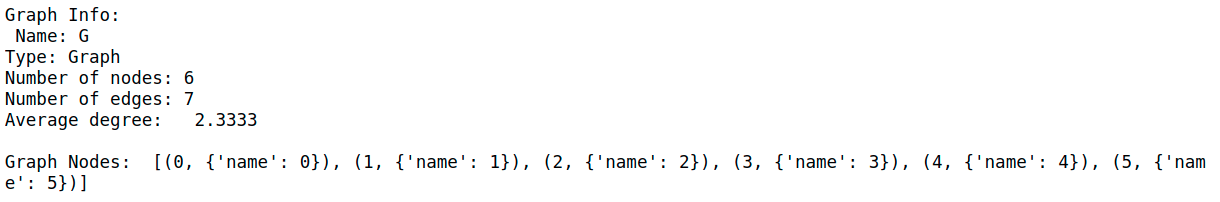Graph G visualization

Since we only have 1 graph, this data configuration is an example of a Single Mode representation. We will build a GCN that will learn the nodes features representation.
由于我们只有1个图，因此此数据配置是“ 单模式”的示例   表示。 我们将构建一个GCN，它将学习节点特征表示。
将邻接矩阵(A)插入正向传递方程 (Inserting Adjacency Matrix (A) to Forward Pass Equation)
The next step is to obtain the Adjacency Matrix (A) and Node Features Matrix (X) from graph G.
下一步是从图G获得邻接矩阵(A)和节点特征矩阵(X) 。
#Get the Adjacency Matrix (A) and Node Features Matrix (X) as numpy array
A = np.array(nx.attr_matrix(G, node_attr='name'))
X = np.array(nx.attr_matrix(G, node_attr='name'))
X = np.expand_dims(X,axis=1)

print('Shape of A: ', A.shape)
print('\nShape of X: ', X.shape)
print('\nNode Features Matrix (X):\n', X)
Output:
输出：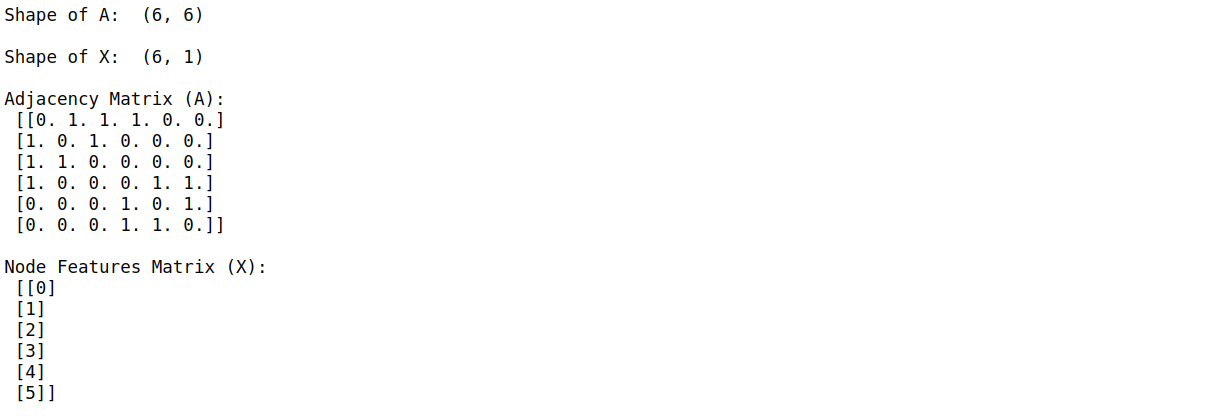Now, let’s investigate how by inserting A into the forward pass equation adds to richer feature representation of the model. We are going to perform dot product of A and X. Let’s call the result of this dot product operation as AX in this article.
现在，让我们研究如何通过将A插入前向传递方程式来增加模型的更丰富的特征表示。 我们将执行A和X的点积。在本文中，我们将此点积运算的结果称为AX 。
#Dot product Adjacency Matrix (A) and Node Features (X)
AX = np.dot(A,X)
print("Dot product of A and X (AX):\n", AX)
Output:
输出：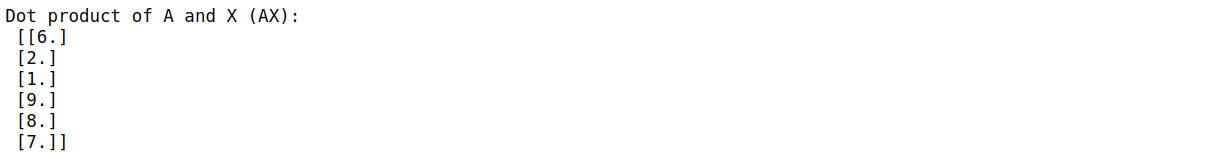From the results, it is apparent that AX represents the sum of neighboring nodes features. For example, the first row of AX corresponds to the sum of nodes features connected to node 0, which is node 1, 2, and 3. This gives us an idea how the propagation mechanism is happening in GCNs and how the node connectivity impacts the hidden features representation seen by GCNs.
从结果可以明显看出AX   表示相邻节点特征的总和 。 例如， AX的第一行对应于连接到节点0(即节点1、2和3)的节点特征之和。这使我们了解了GCN中的传播机制是如何发生的，以及节点的连通性如何影响节点网络。 GCN看到的隐藏特征表示。

The dot product of Adjacency Matrix and Node Features Matrix represents the sum of neighboring node features.
邻接矩阵和节点特征矩阵的点积表示相邻节点特征的总和。

But, if we think about it more, we will realize that while AX sums up the adjacent node features, it does not take into account the features of the node itself.
但是，如果我们考虑得更多，我们将意识到，在AX总结时   相邻节点的特征， 它没有考虑节点本身的特征 。
Oops, problem detected! How to solve it?
糟糕，发现问题！ 怎么解决呢？
插入自环并归一化A (Inserting Self-Loops and Normalizing A)
To address this problem, we now add self-loops to each node of A. Adding self-loops is basically a mechanism to connect a node to itself. That being said, all the diagonal elements of Adjacency Matrix A will now become 1 because each node is connected to itself. Let’s call A with self-loops added as A_hat and recalculate AX, which is now the dot product of A_hat and X:
为了解决这个问题，我们现在向A的每个节点添加自循环 。   自环基本上是一种将节点连接到自身的机制。 就是说，邻接矩阵A的所有对角元素现在将变为1，因为每个节点都与其自身相连。 让我们调用添加了自循环的A作为A_hat并重新计算AX ，它现在是A_hat和X的点积：
#Add Self Loops
G_self_loops = G.copy()

self_loops = []
for i in range(G.number_of_nodes()):
self_loops.append((i,i))

#Check the edges of G_self_loops after adding the self loops
print('Edges of G with self-loops:\n', G_self_loops.edges)

#Get the Adjacency Matrix (A) and Node Features Matrix (X) of added self-lopps graph
A_hat = np.array(nx.attr_matrix(G_self_loops, node_attr='name'))

#Calculate the dot product of A_hat and X (AX)
AX = np.dot(A_hat, X)
print('AX:\n', AX)
Output:
输出：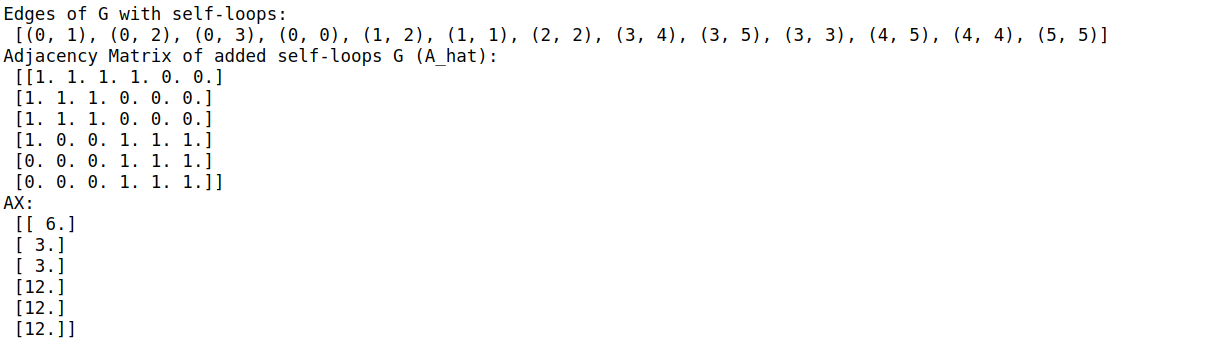Great! One problem solved!
大！ 一个问题解决了！
Now, you might recognize another problem. The elements of AX are not normalized. Similar to data pre-processing for any Neural Networks operation, we need to normalize the features to prevent numerical instabilities and vanishing/exploding gradients in order for the model to converge. In GCNs, we normalize our data by calculating the Degree Matrix (D) and performing dot product operation of the inverse of D with AX
现在，您可能会认识到另一个问题。 AX的元素未规范化 。 类似于任何神经网络操作的数据预处理一样，我们需要对特征进行归一化以防止数值不稳定和消失/爆炸梯度，以使模型收敛。 在GCN中，我们通过计算 度矩阵 (D)并 使用 AX 对D 的 逆 进行点积运算来对数据进行归一化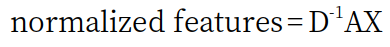which we will call DAX in this article. In graph terminology, the term “degree” refers to the number of edges a node is connected to.
在本文中我们将其称为DAX 。 在图形术语中，术语“度”是指节点连接到的边数。
#Get the Degree Matrix of the added self-loops graph
Deg_Mat = G_self_loops.degree()
print('Degree Matrix of added self-loops G (D): ', Deg_Mat)

#Convert the Degree Matrix to a N x N matrix where N is the number of nodes
D = np.diag([deg for (n,deg) in list(Deg_Mat)])
print('Degree Matrix of added self-loops G as numpy array (D):\n', D)

#Find the inverse of Degree Matrix (D)
D_inv = np.linalg.inv(D)
print('Inverse of D:\n', D_inv)

#Dot product of D and AX for normalization
DAX = np.dot(D_inv,AX)
print('DAX:\n', DAX)
Output:
输出：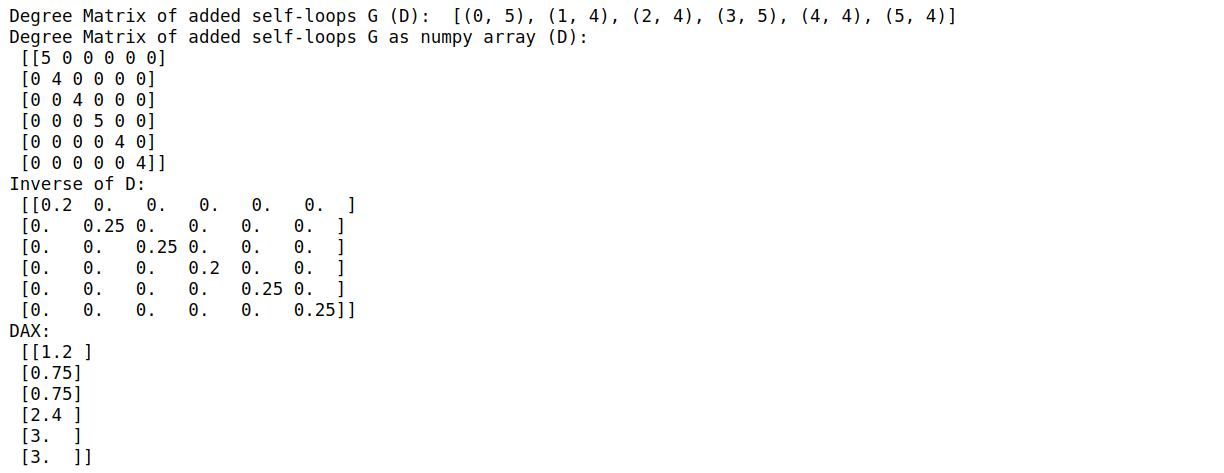If we compare DAX with AX, we will notice that:
如果将DAX与AX进行比较，则会注意到：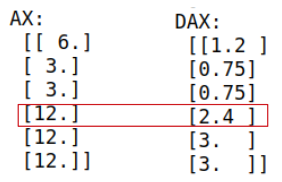We can see the impact normalization has on DAX, where the element that corresponds to node 3 has lower values compared to node 4 and 5. But why would node 3 have different values after normalization if it has the same initial value as node 4 and 5?
我们可以看到归一化对DAX的影响，其中与节点4和5相比，与节点3对应的元素的值较低。但是，如果归一化后节点3与节点4和5的初始值相同，为什么节点3具有不同的值？
Let’s take a look back at our graph. Node 3 has 3 incident edges, while nodes 4 and 5 only have 2 incident edges. The fact that node 3 has a higher degree than node 4 and 5 leads to a lower weighting of node 3’s features in DAX. In other words, the lower the degree of a node, the stronger that a node belongs to a certain group or cluster.
让我们回顾一下我们的图表。 节点3具有3个入射边缘 ，而节点4和5仅具有2个入射边缘 。 节点3具有比节点4和5 高的程度的事实导致DAX中节点3的特征的加权较低 。 换句话说，节点的程度越低，则该节点属于某个组或群集的能力就越强。
In the paper, Kipf and Welling states that doing symmetric normalization will make dynamics more interesting, hence, the normalization equation is modified from:
在Kipf和Welling的论文中 ，进行对称归一化将使动力学更加有趣，因此，对归一化方程进行了以下修改：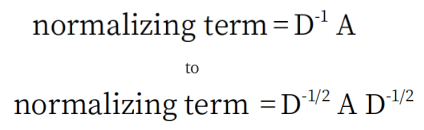Let’s calculate the normalized values using the new symmetric normalization equation:
让我们使用新的对称归一化公式计算归一化值：
#Symmetrically-normalization
D_half_norm = fractional_matrix_power(D, -0.5)
print('DADX:\n', DADX)
Output:
输出：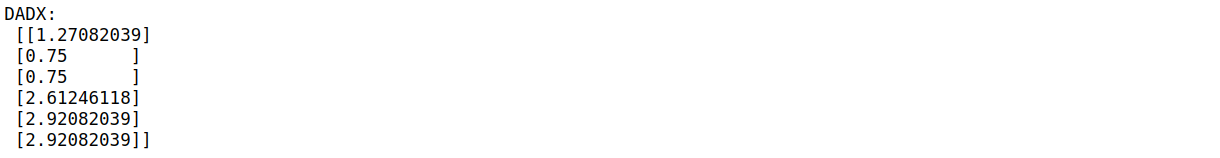Looking back at Equation 3 in the previous section, we will realize that we now have the answers to what is A*! In the paper, A* is referred to as renormalization trick.
回顾上一节中的方程式3，我们将意识到，现在我们有了A *的答案！ 在本文中， A *称为重归一化技巧 。
Having finished with features handling, it’s time to finalize our GCN.
完成功能处理后，是时候完成我们的GCN了。
添加权重和激活功能 (Adding Weights and Activation Function)
We are going to build a 2-layer GCN using ReLu as the activation function. To initialize the weights, we will use random seeds so we can replicate the results. Just keep in mind that the weight initialization cannot be 0. In this experiment, we are going to set 4 neurons for the hidden layer. As we will be plotting the feature representations in 2 dimensions, there will be 2 output neurons.
我们将使用ReLu作为激活功能构建一个2层GCN。 为了初始化权重，我们将使用随机种子，以便我们可以复制结果。 请记住，权重初始化不能为0。在本实验中，我们将为隐藏层设置4个神经元。 由于我们将在2维上绘制特征表示，因此将有2个输出神经元。
Just to make it simpler, we will re-write the renormalization trick equation using numpy, just to make it simpler.
只是为了使其更简单，我们将使用numpy重新编写重归一化技巧方程式，只是为了使其更简单。
#Initialize the weights
np.random.seed(77777)
n_h = 4 #number of neurons in the hidden layer
n_y = 2 #number of neurons in the output layer
W0 = np.random.randn(X.shape,n_h) * 0.01
W1 = np.random.randn(n_h,n_y) * 0.01

#Implement ReLu as activation function
def relu(x):
return np.maximum(0,x)

#Build GCN layer
#In this function, we implement numpy to simplify
def gcn(A,H,W):
I = np.identity(A.shape) #create Identity Matrix of A
A_hat = A + I #add self-loop to A
D = np.diag(np.sum(A_hat, axis=0)) #create Degree Matrix of A
D_half_norm = fractional_matrix_power(D, -0.5) #calculate D to the power of -0.5
eq = D_half_norm.dot(A_hat).dot(D_half_norm).dot(H).dot(W)
return relu(eq)

#Do forward propagation
H1 = gcn(A,X,W0)
H2 = gcn(A,H1,W1)
print('Features Representation from GCN output:\n', H2)
Output:
输出：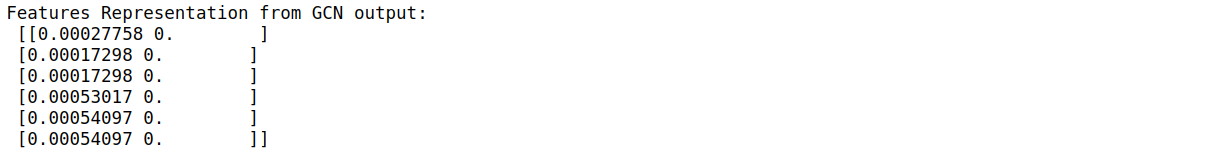Done! We have just built our first feed-forward GCN model!
做完了！ 我们刚刚建立了第一个前馈GCN模型！
绘制特征表示 (Plotting the Features Representations)
The ‘magic’ of GCN is that it can learn features representation even without training. Let’s visualize the features representations after passing through 2-layer GCN.
GCN的“神奇之处”在于， 即使不进行培训 ，它也可以学习特征表示 。 让我们通过2层GCN后可视化要素表示。
def plot_features(H2):
#Plot the features representation
x = H2[:,0]
y = H2[:,1]

size = 1000

plt.scatter(x,y,size)
plt.xlim([np.min(x)*0.9, np.max(x)*1.1])
plt.ylim([-1, 1])
plt.xlabel('Feature Representation Dimension 0')
plt.ylabel('Feature Representation Dimension 1')
plt.title('Feature Representation')

for i,row in enumerate(H2):
str = "{}".format(i)
plt.annotate(str, (row,row),fontsize=18, fontweight='bold')

plt.show()

plot_features(H2)
Output:
输出：
Features Representation from Feed-Forward GCN

From the plot above, it can be clearly seen that there are 2 major groups, where the left group consists of nodes 0, 1, 2, and the right group consists of nodes 3, 4, 5. We can infer that the GCNs can already learn the feature representations even without training or backpropagation.
从上图可以清楚地看到有2个主要组，其中左组由节点0、1、2组成，右组由节点3、4、5组成。我们可以推断出GCN可以即使没有经过培训或 反向传播， 也已经学习了特征表示 。
重要要点 (Key Takeaways)
The term ‘convolution’ in Graph Convolutional Networks is similar to Convolutional Neural Networks in terms of weight sharing. The main difference lies in the data structure, where GCNs are the generalized version of CNN that can work on data with underlying non-regular structures. 在权重分配方面，图卷积网络中的术语“卷积”类似于卷积神经网络。 主要区别在于   数据结构，其中GCN是CNN的通用版本，可以处理具有基础非常规结构的数据。 The insertion of Adjacency Matrix (A) in the forward pass equation of GCNs enable the model to learn the features of neighboring nodes. This mechanism can be seen as a message passing operation along the nodes within the graph. 在GCN的前向传递方程中插入邻接矩阵( A )，使模型可以学习相邻节点的特征。 这种机制可以看作是沿着图内节点的消息传递操作。 Renormalization trick is used to normalize the features in Fast Approximate Spectral-based Graph Convolutional Networks by Thomas Kipf and Max Welling (2017). 重归一化技巧被用于对Thomas Kipf和Max Welling(2017)的基于快速近似谱图的卷积网络中的特征进行归一化。 GCNs can learn features representation even before training. GCN甚至可以在训练之前就学习特征表示。

Thanks for reading! If you want to read about how to train a GCN on node classification task using CORA dataset, you can read the next article in this series.
谢谢阅读！ 如果您想了解如何使用CORA数据集在节点分类任务上训练GCN，可以阅读 本系列 的 下一篇文章 。
Any comment, feedback, or want to discuss? Just drop me a message. You can reach me on LinkedIn.
有任何意见，反馈或要讨论吗？ 请给我留言。 您可以在 LinkedIn上与 我联系 。
You can get the full code on GitHub.
您可以在 GitHub上 获取完整的代码 。

翻译自: https://towardsdatascience.com/understanding-graph-convolutional-networks-for-node-classification-a2bfdb7aba7b

图卷积 节点分类

展开全文人工智能 区块链 机器学习 python java
• 图卷积 节点分类This article goes through the implementation of Graph Convolution Networks (GCN) using Spektral API, which is a Python library for graph deep learning based on Tensorflow 2. We are ...

图卷积 节点分类

This article goes through the implementation of Graph Convolution Networks (GCN) using Spektral API, which is a Python library for graph deep learning based on Tensorflow 2. We are going to perform Semi-Supervised Node Classification using CORA dataset, similar to the work presented in the original GCN paper by Thomas Kipf and Max Welling (2017).
本文介绍了使用 Spektral API 实现图卷积网络(GCN)的情况 ，这是一个基于Tensorflow 2的用于图深度学习的Python库。我们将使用CORA数据集执行半监督节点分类，与所介绍的工作类似在 Thomas Kipf和Max Welling(2017) 的原始GCN论文中 。
If you want to get basic understanding on Graph Convolutional Networks, it is recommended to read the first and the second parts of this series beforehand.
如果您想对图卷积网络有基本的了解，建议您 事先 阅读 本系列 的 第一 和 第二 部分。

数据集概述 (Dataset Overview)
CORA citation network dataset consists of 2708 nodes, where each node represents a document or a technical paper. The node features are bag-of-words representation that indicates the presence of a word in the document. The vocabulary — hence, also the node features — contains 1433 words.
CORA引用网络数据集由2708个节点组成，其中每个节点代表一个文档或技术论文。 节点特征是词袋表示，指示文档中单词的存在。 词汇表-因此，还有节点特征-包含1433个单词。
source
来源说明单词袋作为节点特征

We will treat the dataset as an undirected graph where the edge represents whether one document cites the other or vice versa. There is no edge feature in this dataset. The goal of this task is to classify the nodes (or the documents) into 7 different classes which correspond to the papers’ research areas. This is a single-label multi-class classification problem with Single Mode data representation setting.
我们将数据集视为无向图 ，其中边表示一个文档引用了另一文档，反之亦然。 该数据集中没有边缘特征。 此任务的目标是将节点(或文档)分类为7种不同的类别，分别对应于论文的研究领域。 这是一个单标签多类别分类问题   单模式数据表示设置。
This implementation is also an example of Transductive Learning, where the neural network sees all data, including the test dataset, during the training. This is contrast to Inductive Learning — which is the typical Supervised Learning — where the test data is kept separate during the training.
此实现方式也是Transductive Learning的示例，在训练过程中，神经网络可以查看所有数据，包括测试数据集。 这与归纳学习(典型的监督学习)相反，归纳学习在训练过程中将测试数据保持独立。
文字分类问题 (Text Classification Problem)
Since we are going to classify documents based on their textual features, a common machine learning way to look at this problem is by seeing it as a supervised text classification problem. Using this approach, the machine learning model will learn each document’s hidden representation only based on its own features.
由于我们将根据文档的文本特征对文档进行分类，因此，解决此问题的一种常见的机器学习方法是将其视为有监督的文本分类问题。 使用这种方法，机器学习模型将仅基于自身的功能来学习每个文档的隐藏表示。
Illustration of text classification approach on a document classification problem (image by author)

This approach might work well if there are enough labeled examples for each class. Unfortunately, in real world cases, labeling data might be expensive.
如果每个类都有足够的带标签的示例，则此方法可能会很好用。 不幸的是，在现实情况下，标记数据可能会很昂贵。
What is another approach to solve this problem?
解决此问题的另一种方法是什么？
Besides its own text content, normally, a technical paper also cites other related papers. Intuitively, the cited papers are likely to belong to similar research area.
除了自身的文本内容外，技术论文通常还会引用其他相关论文。 从直觉上讲，被引论文可能属于相似的研究领域。
In this citation network dataset, we want to leverage the citation information from each paper in addition to its own textual content. Hence, the dataset has now turned into a network of papers.
在这个引文网络数据集中，我们希望利用每篇论文的引文信息以及自己的文本内容。 因此，数据集现在变成了论文网络。
Illustration of citation network dataset with partly labeled data (image by author)

Using this configuration, we can utilize Graph Neural Networks, such as Graph Convolutional Networks (GCNs), to build a model that learns the documents interconnection in addition to their own textual features. The GCN model will learn the nodes (or documents) hidden representation not only based on its own features, but also its neighboring nodes’ features. Hence, we can reduce the number of necessary labeled examples and implement semi-supervised learning utilizing the Adjacency Matrix (A) or the nodes connectivity within a graph.
使用此配置，我们可以利用诸如图卷积网络(GCN)之类的图神经网络来构建一个模型，该模型除了学习其自身的文本特征外，还可以学习文档的互连。 GCN模型将不仅基于其自身的特征，而且还基于其邻近节点的特征，来学习节点(或文档)的隐藏表示。 因此，我们可以减少必要的带标签示例的数量，并利用邻接矩阵(A)进行半监督学习   或图中的节点连通性。
Another case where Graph Neural Networks might be useful is when each example does not have distinct features on its own, but the relations between the examples can enrich the feature representations.
图神经网络可能有用的另一种情况是，每个示例自身都不具有明显的特征，但是示例之间的关系可以丰富特征表示。

图卷积网络的实现 (Implementation of Graph Convolutional Networks)
In this experiment, we are going to build and train a GCN model using Spektral API that is built on Tensorflow 2. Although Spektral provides built-in functions to load and preprocess CORA dataset, in this article we are going to download the raw dataset from here in order to gain deeper understanding on the data preprocessing and configuration. The complete code of the whole exercise in this article can be found on GitHub.
在此实验中，我们将使用基于Tensorflow 2构建的Spektral API来构建和训练GCN模型。尽管Spektral提供了内置功能来加载和预处理CORA数据集，但在本文中，我们将从以下位置下载原始数据集： 在这里 ，以获得对数据预处理和配置更深入的了解。 本文整个练习的完整代码可以在GitHub上找到 。
We use cora.content and cora.cites files in the respective data directory. After loading the files, we will randomly shuffle the data.
我们在各自的数据目录中使用cora.content和cora.cites文件。 加载文件后，我们将随机重新整理数据。

In cora.content file, each line consists of several elements:the first element indicates the document (or node) ID,the second until the last second elements indicate the node features,the last element indicates the label of that particular node.
在cora.content文件中，每一行包含几个元素：第一个元素指示文档(或节点)ID， 第二个直到最后一个第二元素指示节点特征， 最后一个元素指示该特定节点的标签。
In cora.cites file, each line contains a tuple of documents (or nodes) IDs. The first element of the tuple indicates the ID of the paper being cited, while the second element indicates the paper containing the citation. Although this configuration represents a directed graph, in this approach we treat the dataset as an undirected graph.
在cora.cites文件中，每行包含一个文档(或节点)ID的元组。 元组的第一个元素指示被引用论文的ID ，而第二个元素指示包含被引用论文 。 尽管此配置表示有向图，但是在这种方法中，我们将数据集视为无向图 。
After loading the data, we build Node Features Matrix (X) and a list containing tuples of adjacent nodes. This edges list will be used to build a graph from where we can obtain the Adjacency Matrix (A).
加载数据后，我们构建节点特征矩阵( X )和一个包含相邻节点元组的列表。 此边缘列表将用于构建图，从中可以获取邻接矩阵( A )。

Output:
输出：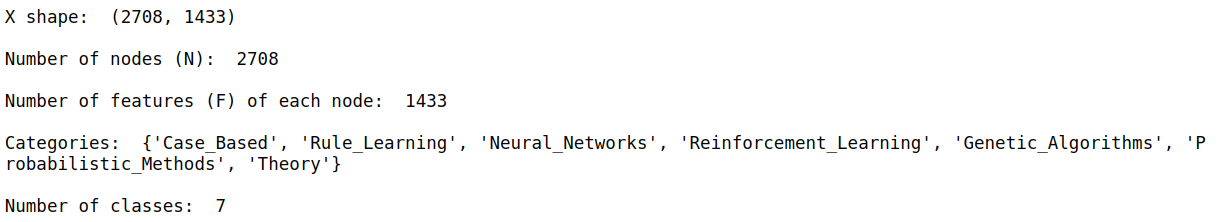设置训练，验证和测试掩码 (Setting the Train, Validation, and Test Mask)
We will feed in the Node Features Matrix (X) and Adjacency Matrix (A) to the neural networks. We are also going to set Boolean masks with a length of N for each training, validation, and testing dataset. The elements of those masks are True when they belong to corresponding training, validation, or test dataset. For example, the elements of train mask are True for those which belong to training data.
我们将节点特征矩阵( X )和邻接矩阵( A )馈入神经网络。 我们还将为每个设置长度为N的 布尔掩码   训练，验证和测试数据集。 这些遮罩的元素属于相应的训练，验证或测试数据集时，它们为True 。 例如，对于属于训练数据的那些元素，训练蒙版的元素为True 。
Examples of Train, Validation, and Test Boolean Masks

In the paper, they pick 20 labeled examples for each class. Hence, with 7 classes, we will have a total of 140 labeled training examples. We will also use 500 labeled validation examples and 1000 labeled testing examples.
在本文中，他们为每个班级选取20个带有标签的示例。 因此，通过7个课程，我们将总共有140个带有标签的培训示例。 我们还将使用500个带标签的验证示例和1000个带标签的测试示例。

The next step is to obtain the Adjacency Matrix (A) of the graph. We use NetworkX to help us do this. We will initialize a graph and then add the nodes and edges lists to the graph.
下一步是获取图的邻接矩阵( A )。 我们使用NetworkX来帮助我们做到这一点。 我们将初始化一个图，然后将节点和边列表添加到图中。

Output:
输出：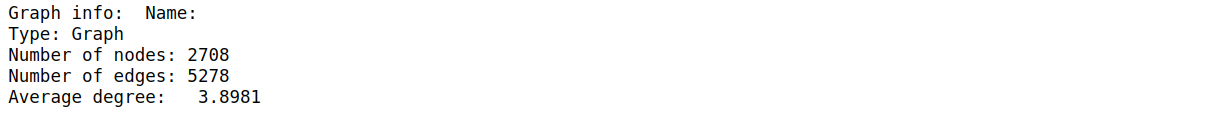将标签转换为一键编码 (Converting the label to one-hot encoding)
The last step before building our GCN is, just like any other machine learning model, encoding the labels and then converting them to one-hot encoding.
与其他任何机器学习模型一样，构建GCN之前的最后一步是对标签进行编码，然后将其转换为一次性编码。

We are now done with data preprocessing and ready to build our GCN!
现在，我们已经完成了数据预处理，并准备构建我们的GCN！
建立图卷积网络 (Build the Graph Convolutional Networks)
The GCN model architectures and hyperparameters follow the design from GCN original paper. The GCN model will take 2 inputs, the Node Features Matrix (X) and Adjacency Matrix (A). We are going to implement 2-layer GCN with Dropout layers and L2 regularization. We are also going to set the maximum training epochs to be 200 and implement Early Stopping with patience of 10. It means that the training will be stopped once the validation loss does not decrease for 10 consecutive epochs. To monitor the training and validation accuracy and loss, we are also going to call TensorBoard in the callbacks.
GCN模型的体系结构和超参数遵循GCN原始论文的设计。 GCN模型将采用2个输入，即节点特征矩阵( X )和邻接矩阵( A )。 我们将使用 Dropout层和 L2正则化实现2层GCN 。 我们还将最大训练时间设为200，并以10的耐心实施“ 提前停止” 。 这意味着一旦验证损失连续10个周期没有减少，训练就会停止。 为了监控训练和验证的准确性和损失，我们还将在回调中调用TensorBoard 。
Before feeding in the Adjacency Matrix (A) to the GCN, we need to do extra preprocessing by performing renormalization trick according to the original paper. You can also read about how renormalization trick affects GCN forward propagation calculation here.
在将邻接矩阵( A )输入到GCN之前，我们需要根据原始论文通过执行重新规范化技巧来进行额外的预处理。 您还可以阅读有关重归一化技巧如何影响GCN前向传播计算的信息   在这里 。
The code to train GCN below was originally obtained from Spektral GitHub page.
下面训练GCN的代码最初是从Spektral GitHub页面获得的。

训练图卷积网络 (Train the Graph Convolutional Networks)
We are implementing Transductive Learning, which means we will feed the whole graph to both training and testing. We separate the training, validation, and testing data using the Boolean masks we have constructed before. These masks will be passed to sample_weight argument. We set the batch_size to be the whole graph size, otherwise the graph will be shuffled.
我们正在实施“归纳式学习”，这意味着我们将把整个图表馈送给培训和测试。 我们使用之前构造的布尔掩码将训练，验证和测试数据分开。 这些掩码将传递给sample_weight参数。 我们将batch_size设置为整个图的大小，否则该图将被重新排序。
To better evaluate the model performance for each class, we use F1-score instead of accuracy and loss metrics.
为了更好地评估每个类别的模型性能，我们使用F1评分而不是准确性和损失指标。

Training done!
培训完成！
From the classification report, we obtain macro average F1-score of 74%.
从分类报告中，我们获得74％的宏观平均F1得分。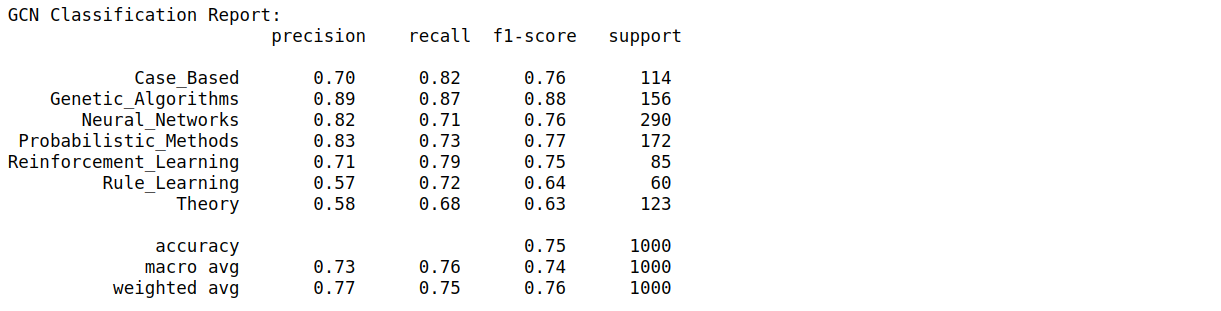使用t-SNE的隐藏层激活可视化 (Hidden Layers Activation Visualization using t-SNE)
Let’s now use t-SNE to visualize the hidden layer representations. We use t-SNE to reduce the dimension of the hidden representations to 2-D. Each point in the plot represents each node (or document), while each color represents each class.
现在让我们使用t-SNE可视化隐藏层表示。 我们使用t-SNE将隐藏表示的尺寸减小为2D。 图中的每个点代表每个节点(或文档)，而每种颜色代表每个类别。

Output:
输出：
T-SNE Representation of GCN Hidden Layer. GCN is able to learn features representations considerably well, shown by distinct data clusters.

与完全连接的神经网络的比较 (Comparison to Fully Connected Neural Networks)
As a benchmark, I also trained a 2-layer Fully Connected Neural Networks (FCNN) and plot the t-SNE visualization of hidden layer representations. The results are shown below:
作为基准，我还训练了2层全连接神经网络(FCNN)，并绘制了隐藏层表示的t-SNE可视化。 结果如下所示：
Classification Results from 2-layer Fully Connected Neural Networks

T-SNE Representation of FCNN Hidden Layer Representation. FCNN cannot classify the documents as well as GCN.

From the results above, it is clear that GCN significantly outperforms FCNN with macro average F1-score is only 55%. The t-SNE visualization plot of FCNN hidden layer representations is scattered, which means that FCNN can’t learn the features representations as well as GCN.
从以上结果可以明显看出，GCN的性能明显优于FCNN，宏观平均F1得分仅为55％。 FCNN隐藏层表示的t-SNE可视化图是分散的，这意味着FCNN无法像GCN一样学习特征表示。
结论 (Conclusion)
The conventional machine learning approach to perform document classification, for example CORA dataset, is to use supervised text classification approach. Graph Convolutional Networks (GCNs) is an alternative semi-supervised approach to solve this problem by seeing the documents as a network of related papers. Using only 20 labeled examples for each class, GCNs outperform Fully-Connected Neural Networks on this task by around 20%.
执行文档分类的常规机器学习方法(例如CORA数据集)是使用监督文本分类方法。 图卷积网络(GCN)是通过将文档视为相关论文的网络来解决此问题的另一种半监督方法。 对于每个类别，仅使用20个带有标签的示例，GCN在此任务上的性能就比全连接神经网络高出约20％。
谢谢阅读！ 我希望本文能帮助您针对自己的问题实现图卷积网络(GCN)。

Any comment, feedback, or want to discuss? Just drop me a message. You can reach me on LinkedIn.
有任何意见，反馈或要讨论吗？ 请给我留言。 您可以在 LinkedIn上与 我联系 。
You can find the full code on GitHub.
您可以在 GitHub上 找到完整的代码 。

翻译自: https://towardsdatascience.com/graph-convolutional-networks-on-node-classification-2b6bbec1d042

图卷积 节点分类

展开全文机器学习 人工智能 算法 深度学习 python
• 数据聚类，主要对于数据点进行聚类，输出节点分类情况和节点中心
• 在图谱当中，有一项很重要的任务，节点分类。该任务通常是给定图中某些节点对应的类别，从而预测出生于没有标签的节点属于哪一个类别，该任务也被称为半监督节点分类。 本文主要要解决的问题就是如何做节点分类。 图...
前言
在图谱当中，有一项很重要的任务，节点分类。该任务通常是给定图中某些节点对应的类别，从而预测出生于没有标签的节点属于哪一个类别，该任务也被称为半监督节点分类。
本文主要介绍三种图算法来解决节点分类问题。
图中的相互关系
在图谱中，存在着两种重要的相互关系
homophily亲和性(我自己的翻译成，不一定准确)，具体意思就是指人以群分物以类聚，例如在社交网络中，喜欢蔡徐坤的人通常都会有同样的喜好。influence影响性，某节点的行为可能会影响到和他有关系的节点行为，例如有一天你吃起了螺蛳粉，结果你身边的人都跟着你吃了起来。
那么，如何利用这些关系来预测节点的标签呢？
通常，相似的节点都会紧密相连或者直接相连，而相连的节点大概率会有相同的标签。例如非法网站，通常都会有其它非法网站的链接。因此，我们预测节点类别时，通常会注意以下三个方面信息：
目标节点特征目标节点的邻居节点的labels目标节点的邻居节点的特征
有了以上的概念，我们就具体来看下有哪些节点分类的方法。注意，以下算法都遵循马尔科夫假设，即节点i的标签只是其邻居节点的标签有关系。
Probabilistic Relational Classifier概率关系分类器
基本思想：某节点的label是其邻居节点的对应的label概率的均值。
首先初始化已经存在label的节点标签概率，正例是1，负例是0，对于没有标签的全部设置为0.5，然后对所有没有标签的节点进行概率更新，直到收敛或者得到最大的迭代次数。（感觉是一个马尔科夫过程）

P

(

Y

i

=

c

)

=

1

∑

(

i

,

j

)

∈

E

W

(

i

,

j

)

∑

(

i

,

j

)

∈

E

W

(

i

,

j

)

P

(

Y

j

=

c

)

P(Y_i=c)=\frac{1}{\sum_{(i,j) \in E}W(i,j)}\sum_{(i,j)\in E}W(i,j)P(Y_j=c)

其中

W

(

i

,

j

)

W(i,j)

表示的是节点

i

i

与节点

j

j

的边的权重。
接下来我们来看一个具体的例子：
初始化所有节点的概率值，没有标签的节点采用均匀分布设置为0.5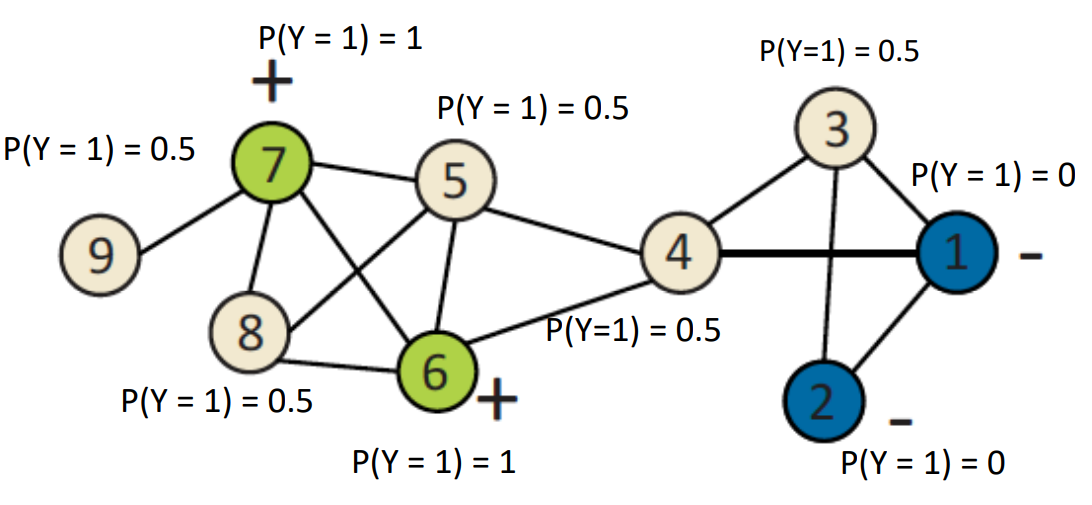对节点3进行新的概率更新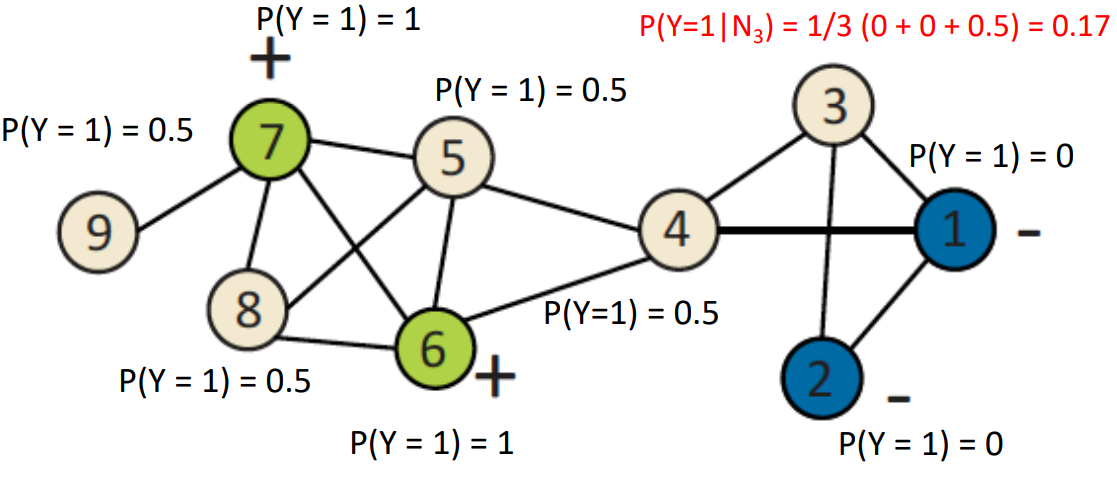对节点4进行概率更新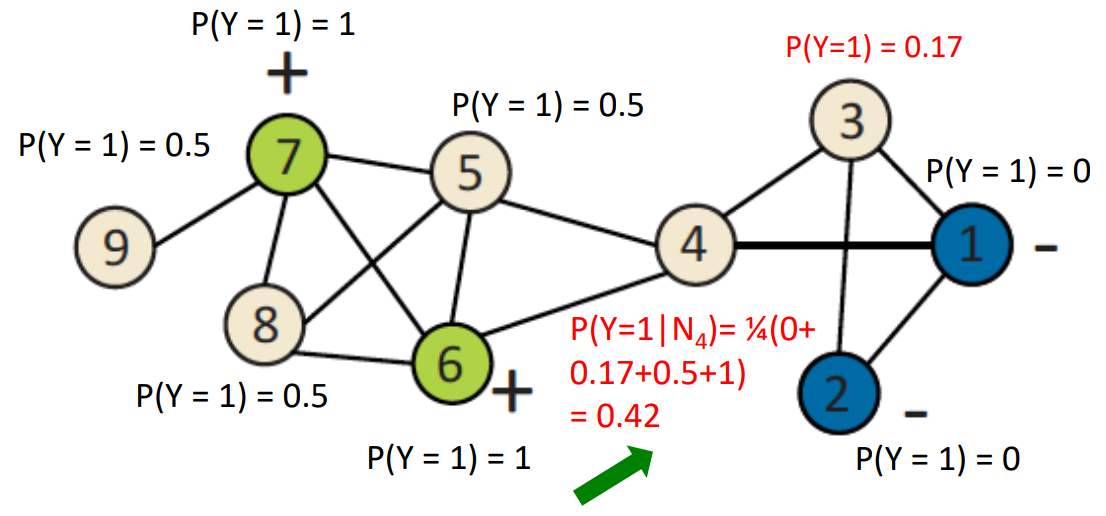迭代一轮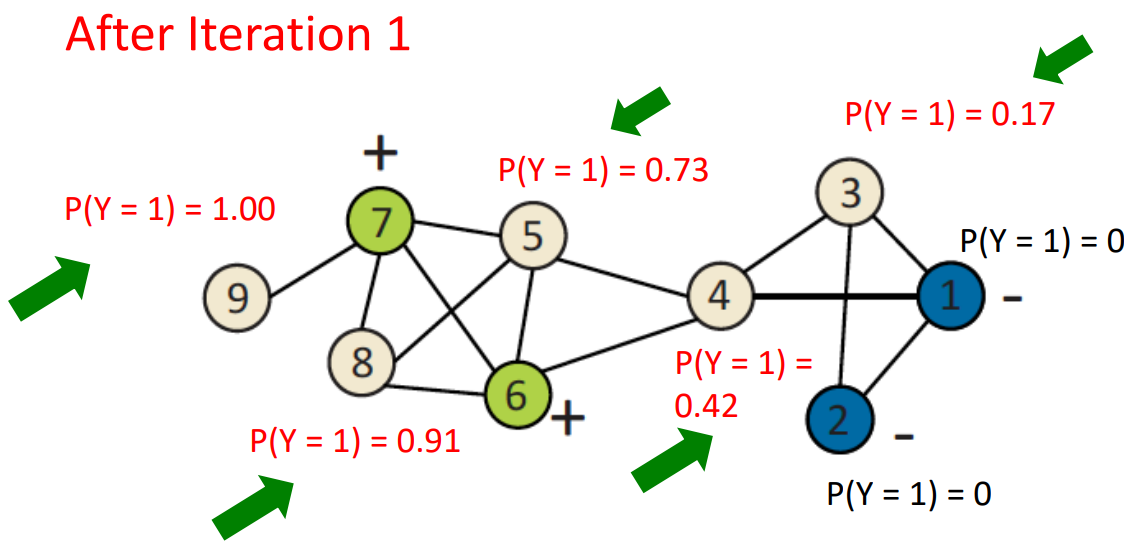迭代五轮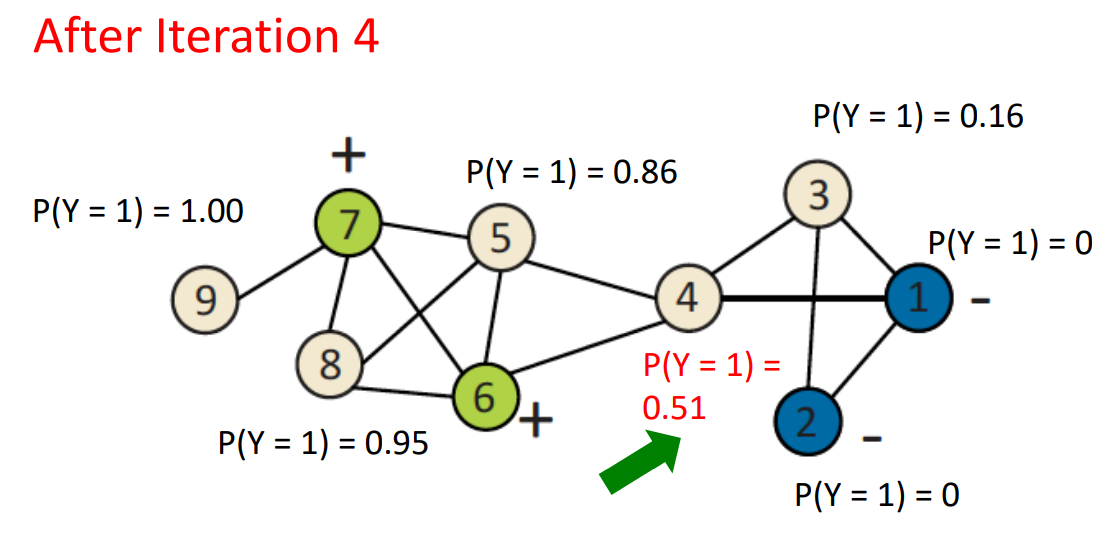五轮迭代后，所有的概率值都趋于稳定，此时节点5、8、9对应的概率值大于0.5，设置为正例，节点3概率值小于0.5设置为负例，节点4概率值趋于0.5则正负都有可能。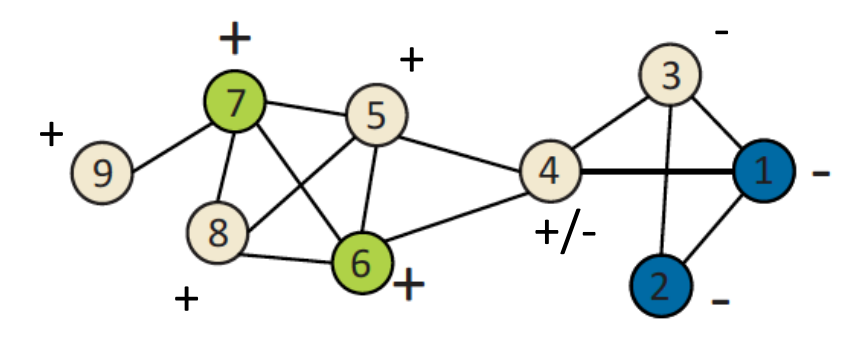缺点:
收敛难以得到保障（节点4）没有利用节点的特征信息
Iterative Classification迭代分类
Iterative classification实际上就是考虑关系的同时也考虑节点的的属性，主要包括三点
对于节点

i

i

，创建一个向量

a

i

a_i

使用

a

i

a_i

来训练分类器(例如LR、SVM等)如果一个节点有多个邻居节点，做一个聚合操作，计算其数量，众数，比例，均值，是否存在邻居等。
训练过程和上一个算法类似，不停的迭代更新每一个节点的label，注意因为节点的改变，对应的节点的向量

a

i

a_i

也需要更新。知道label稳定，或者达到最大的迭代次数，训练结束。
缺点： 该算法的收敛依旧没有得到保证。
Belief Propagation信念传播
Belief Propagation信念传播简称BP，是一种在图中通过计算条件概率的形式来表示消息传递的算法，可以理解为马尔科夫随机场，该算法采用了动态规划。
在开始之前，我们先了解几个概念：
message：message表示的是从节点

i

i

到节点

j

j

传递的信息，通常表示为

m

i

→

j

(

X

j

)

m_{i\to j}(X_j)

，message和概率很类型，非负但是其和不是1，如果

m

i

→

j

(

X

j

)

m_{i\to j}(X_j)

越高，说明边缘概率

P

(

X

j

)

P(X_j)

的值越高，通常message的初始值会设置为1belief：边缘概率即被称为belief
BP算法实际上就是不停的迭代更新message直到收敛再计算belief。看个具体的例子，如下图，我们想知道

k

k

到底传递给了

j

j

什么信息。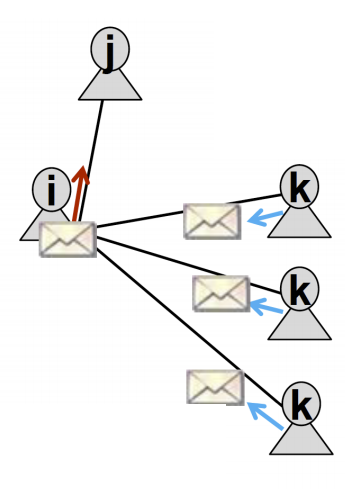m

i

→

j

(

Y

j

)

m_{i \to j}(Y_j)

即上文提到的message，可以理解为是在计算整个图的联合概率，所以有如下公式：

m

i

→

j

(

Y

j

)

=

α

∑

Y

i

∈

L

ψ

(

Y

i

,

Y

j

)

ϕ

i

(

Y

i

)

∏

k

∈

N

i

∖

j

m

k

→

i

(

Y

i

)

m_{i \to j}(Y_j)=\alpha \sum_{Y_i \in \mathcal L} \psi(Y_i,Y_j) \phi_i(Y_i) \prod_{k \in N_i \setminus j}m_{k \to i(Y_i)}

解释一下这个公式，

∑

Y

i

∈

L

\sum_{Y_i \in \mathcal L}

表示的是对所有状态求和，

ψ

(

Y

i

,

Y

j

)

\psi(Y_i,Y_j)

是状态转移概率，表示的是已知节点

j

j

的邻居节点

i

i

的状态

Y

i

Y_i

，

j

j

节点状态为

Y

j

Y_j

的概率，可以理解为条件概率

ϕ

i

(

Y

i

)

\phi_i(Y_i)

表示的是节点

i

i

状态为

Y

i

Y_i

的概率，可以理解为先验概率

L

\mathcal L

表示的是所有状态的集合
上图只是一个比较简单的图，如果图比较复杂，那么就随机在图中选择一个节点作为根节点，然后从叶节点开始传递消息，重复这个过程n次，直到模型收敛。注意，每次消息传递的过程message的值都会保存下来，这就是算法中的动态规划。
因为每个结点都会收到来⾃所有相邻结点的信息，因此就可以计算每个节点的边缘概率即belief

b

i

(

Y

i

)

=

α

ϕ

i

(

Y

i

)

∏

j

∈

N

i

m

i

→

j

(

Y

i

)

，

∀

Y

i

∈

L

b_i(Y_i)=\alpha \phi_i(Y_i) \prod_{j \in N_i }m_{i \to j(Y_i)}，\forall Y_i \in \mathcal L

边缘概率最高的对应的类别就是当前节点的所属类别。
BP可以并行的进行计算，所以效率很高，但是该算法依旧没办法完全保证模型收敛，特别是有环的时候。
总结
本文介绍的节点分类方法都是基于传统的图算法，目前也有很多基于Node Vector、GNN的方法来做node classification，相关的博文我会尽快分享给大家，敬请期待。
References
cs224w 6. Message Passing and Node Classification
展开全文知识图谱
• 图网络算法——信息传递和节点分类 在开始介绍下面的算法问题之前，我们首先从提出一个问题，给定一个某些节点具有分类标签的网络结构，我们应该如何去预测网络中其他节点的标签呢？ 这种节点分类的方式称为半监督...
• 之前的笔记【学习笔记】图神经网络库 DGL 入门教程（backend pytorch） 写得比较详尽，但是教程中的代码写得比较零散，这里抽空把两个最常见的任务，节点分类和边分类的代码整合了一下，加了一些注释便于理解，已备...深度学习
• 文中在节点分类和图分类任务上进行了实验，结果表明，graph U-Nets在transductive learning任务上比以前的GNNs取得了更好的性能。 为了避免采样图中可能存在的孤立节点问题，文中采用图2次幂来改进了图的连通性。 ...
• ## 比特币节点分类

千次阅读 2020-01-31 22:31:55
比特币网络指的就是运行了比特币 P2P 协议的很多节点的集合，每个节点地位上都是平等的，但是由于侧重的功能不同，其实比特币节点是分不同的角色的。 节点功能 钱包，指的是钱包软件，而非地址加私钥本身。钱包的...区块链 比特币
• 为确保对等网络节点交互的安全性，提出一种基于交易节点分类管理的网络安全模型。将失败的交易分为严重失败与一般不满意进行分类统计，以便更准确及时地检测恶意节点。在节点的直接交易过程中，根据交易历史记录，...
• 1. 比特币中的节点分类 保存自身的交易信息以及数据保存自身的交易信息比特币节点分类全节点维持包含全部交易信息的完整区块链的节点轻节点SPV节点仍需下载全部数据进行解析,获取与自身相关的交易数据,但无须本地...
• 评估图嵌入H（n*d矩阵）在节点分类任务上的表现，通过逻辑回归以H为输入训练分类器，10折交叉验证 代码如下 在这里插入from sklearn.multiclass import OneVsRestClassifier from sklearn.linear_model import ...数据挖掘Python
• 基于此，提出一种新的基于能量感知的节点分类控制路由算法（CEAR），算法首先通过定义的能量阈值计算公式，周期性地将普通节点分为骨干节点和独立节点，因而保护了能量低的节点；然后两类节点通过设计的骨干路由树和...
• Fabric 节点分类以及配置节点分类Endorsing PeerCommitting PeerAnchor PeerLeading PeerOrderer Peer（O_S） 节点分类 在 HF 中，有背书节点，提交节点，锚节点和领导节点。一个节点可以同时是一个提交节点，背书...区块链 分布式
• 文章目录Abstract1.Intruduction2.Fast Approximate Convolutions on Graphs（图的快速近似卷积）2.1 SpectralGraph Convolutins（谱图卷积）补充证明... Semi-supervised Node Classfication（半监督节点分类）3.1...图卷积 Graph
• 文章目录 基准图数据 Cora数据集 用GCN进行半监督节点分类 基准图数据 Pythorch Geometric还包含大量常见的基准数据集，例如Planetoid数据集（包含Cora、Citeseer、Pubmed三个子数据集），来自... 初始化数据集很简单。...图神经网络 pytorch
• 介绍了HTML DOM节点分类以及各类别的基本知识~javascript
• 1、Arithmetic 计算节点 2、Constant Vectors 常数向量节点 一维、二维向量、矩阵 3、Properties 属性节点 4、Vector Operations 属性节点 向量操作 计算向量长度、将向量标准化、计算一个向量到另一个向量的...Shader
• 主要介绍了PHP带节点操作的无限分类实现方法,可实现无限分类及针对节点的添加、删除、移动等功能,需要的朋友可以参考下
• ## Elasticsearch节点类型

千次阅读 2019-06-17 20:54:52
Elasticsearch2.x节点类型 节点类型 中文名称 配置 作用 备注 master节点节点 node.master=truenode.data=false
• Zookeeper的节点分类 总体来说，Znode节点可以分为以下四类。 一个Znode节点可以是持久性的，也可以是临时性的。 持久性的Znode：创建节点后即使Zookeeper集群宕机，或者Zookeeper客户端宕机，节点也不会丢失。 ...
• ## 节点类型

千次阅读 2018-09-27 15:40:17
前面提到默认情况下节点既可以做候选主节点也可以做数据节点，但是数据节点的负载较重，所以需要考虑将二者分离开，设置专用的数据节点，避免因数据节点负载重导致主节点不响应。 node . master = false ......